# Lecture 7

23. Mar 2019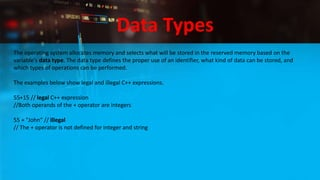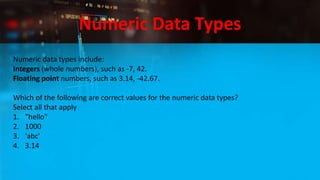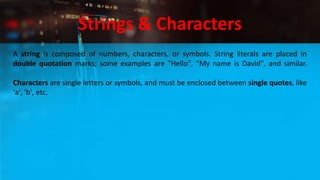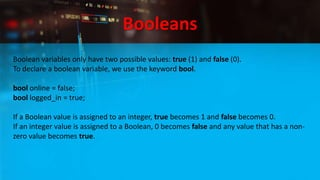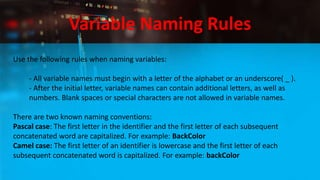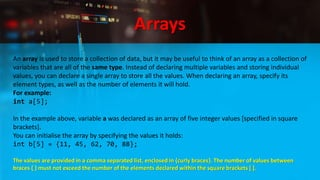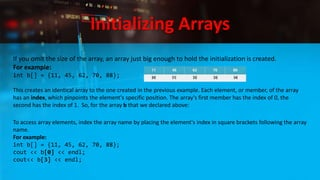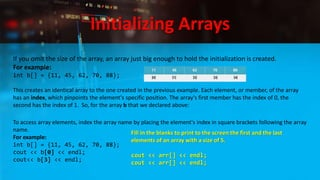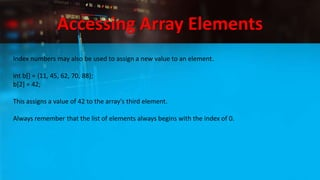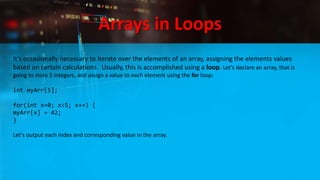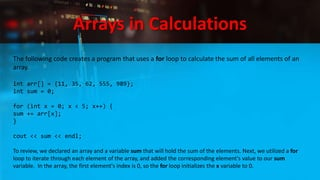1 von 16

### Lecture 7

• 1. C++ Programming Data Types, Arrays, Pointers Mohammed Jehan Lecturer
• 2. Data Types The operating system allocates memory and selects what will be stored in the reserved memory based on the variable's data type. The data type defines the proper use of an identifier, what kind of data can be stored, and which types of operations can be performed. The examples below show legal and illegal C++ expressions. 55+15 // legal C++ expression //Both operands of the + operator are integers 55 + "John" // illegal // The + operator is not defined for integer and string
• 3. Numeric Data Types Numeric data types include: Integers (whole numbers), such as -7, 42. Floating point numbers, such as 3.14, -42.67. Which of the following are correct values for the numeric data types? Select all that apply 1. "hello" 2. 1000 3. 'abc' 4. 3.14
• 4. Strings & Characters A string is composed of numbers, characters, or symbols. String literals are placed in double quotation marks; some examples are "Hello", "My name is David", and similar. Characters are single letters or symbols, and must be enclosed between single quotes, like 'a', 'b', etc.
• 5. Signed and unsigned Integers Use the int keyword to define the integer data type.int a = 42; Several of the basic types, including integers, can be modified using one or more of these type modifiers: signed: A signed integer can hold both negative and positive numbers. unsigned: An unsigned integer can hold only positive values. short: Half of the default size. long: Twice the default size. For example: unsigned long int a; NOTE: The size of the integer type varies according to the architecture of the system on which the program runs, although 4 bytes is the minimum size in most modern system architectures.
• 6. Floating Point Numbers A floating point type variable can hold a real number, such as 420.0, -3.33, or 0.03325. The words floating point refer to the fact that a varying number of digits can appear before and after the decimal point. You could say that the decimal has the ability to "float". There are three different floating point data types: float, double, and long double. In most modern architectures, a float is 4 bytes, a double is 8, and a long double can be equivalent to a double (8 bytes), or 16 bytes. For example: double temp = 4.21; Floating point data types are always signed, which means that they have the capability to hold both positive and negative values.
• 7. Strings A string is an ordered sequence of characters, enclosed in double quotation marks. It is part of the Standard Library. You need to include the <string> library to use the string data type. Alternatively, you can use a library that includes the string library. #include <string> using namespace std; int main() { string a = "I am learning C++"; return 0; }
• 8. Characters A char variable holds a 1-byte integer. However, instead of interpreting the value of the char as an integer, the value of a char variable is typically interpreted as an ASCII character. A character is enclosed between single quotes (such as 'a', 'b', etc). For example: char test = 'S'; American Standard Code for Information Interchange (ASCII) is a character-encoding scheme that is used to represent text in computers.
• 9. Booleans Boolean variables only have two possible values: true (1) and false (0). To declare a boolean variable, we use the keyword bool. bool online = false; bool logged_in = true; If a Boolean value is assigned to an integer, true becomes 1 and false becomes 0. If an integer value is assigned to a Boolean, 0 becomes false and any value that has a non- zero value becomes true.
• 10. Variable Naming Rules Use the following rules when naming variables: - All variable names must begin with a letter of the alphabet or an underscore( _ ). - After the initial letter, variable names can contain additional letters, as well as numbers. Blank spaces or special characters are not allowed in variable names. There are two known naming conventions: Pascal case: The first letter in the identifier and the first letter of each subsequent concatenated word are capitalized. For example: BackColor Camel case: The first letter of an identifier is lowercase and the first letter of each subsequent concatenated word is capitalized. For example: backColor
• 11. Arrays An array is used to store a collection of data, but it may be useful to think of an array as a collection of variables that are all of the same type. Instead of declaring multiple variables and storing individual values, you can declare a single array to store all the values. When declaring an array, specify its element types, as well as the number of elements it will hold. For example: int a; In the example above, variable a was declared as an array of five integer values [specified in square brackets]. You can initialise the array by specifying the values it holds: int b = {11, 45, 62, 70, 88}; The values are provided in a comma separated list, enclosed in {curly braces}. The number of values between braces { } must not exceed the number of the elements declared within the square brackets [ ].
• 12. Initializing Arrays If you omit the size of the array, an array just big enough to hold the initialization is created. For example: int b[] = {11, 45, 62, 70, 88}; This creates an identical array to the one created in the previous example. Each element, or member, of the array has an index, which pinpoints the element's specific position. The array's first member has the index of 0, the second has the index of 1. So, for the array b that we declared above: To access array elements, index the array name by placing the element's index in square brackets following the array name. For example: int b[] = {11, 45, 62, 70, 88}; cout << b << endl; cout<< b << endl;
• 13. Initializing Arrays If you omit the size of the array, an array just big enough to hold the initialization is created. For example: int b[] = {11, 45, 62, 70, 88}; This creates an identical array to the one created in the previous example. Each element, or member, of the array has an index, which pinpoints the element's specific position. The array's first member has the index of 0, the second has the index of 1. So, for the array b that we declared above: To access array elements, index the array name by placing the element's index in square brackets following the array name. For example: int b[] = {11, 45, 62, 70, 88}; cout << b << endl; cout<< b << endl; Fill in the blanks to print to the screen the first and the last elements of an array with a size of 5. cout << arr[] << endl; cout << arr[] << endl;
• 14. Accessing Array Elements Index numbers may also be used to assign a new value to an element. int b[] = {11, 45, 62, 70, 88}; b = 42; This assigns a value of 42 to the array's third element. Always remember that the list of elements always begins with the index of 0.
• 15. Arrays in Loops It's occasionally necessary to iterate over the elements of an array, assigning the elements values based on certain calculations. Usually, this is accomplished using a loop. Let's declare an array, that is going to store 5 integers, and assign a value to each element using the for loop: int myArr; for(int x=0; x<5; x++) { myArr[x] = 42; } Let's output each index and corresponding value in the array.
• 16. Arrays in Calculations The following code creates a program that uses a for loop to calculate the sum of all elements of an array. int arr[] = {11, 35, 62, 555, 989}; int sum = 0; for (int x = 0; x < 5; x++) { sum += arr[x]; } cout << sum << endl; To review, we declared an array and a variable sum that will hold the sum of the elements. Next, we utilized a for loop to iterate through each element of the array, and added the corresponding element's value to our sum variable. In the array, the first element's index is 0, so the for loop initializes the x variable to 0.